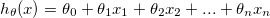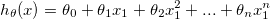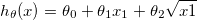## 多项式拟合(Polynomial Regression)

Linear regression:``````h = theta0 + theta1x1 + theta2x2 + ... + theta3x3
``````

Polynomial regression:``````h = theta0 + theta1x1 + theta2x1^2 + ... + thetanx1^n
let x2 = x1^2, xn = x1^n, then,
h = theta0 + theta1x1 + theta2x2 + ... thetanxn # same as linear regression.
``````

or,``````h = theta0 + theta1x1 + theta2sqrt(x1)
let x2 = sqrt(x1), then,
h = theta0 + theta1x1 + theta2x2 # same as linear regression.
``````

## 特征归一化(Feature normalization)

• Feature scaling
• Mean normalization.

### Feature scaling

``````xj = （xj - u) / (xmax - xmin)
``````

### Mean normalization

``````xj = (xj - u) / std,  std为标准差
``````